# Gibb's paradox and its resolution

Gibbs' paradox is a problem in statistical mechanics that arises when attempting to calculate the entropy of a mixture of two ideal gases. The paradox is that the entropy of the mixture is greater than the sum of the entropies of the individual gases, which seems to violate the second law of thermodynamics.

The resolution to Gibbs' paradox lies in the fact that the individual gas particles are not distinguishable from each other, while the particles in the mixture are. When calculating the entropy of the mixture, the number of possible arrangements of particles increases due to the distinguishability of the particles. This increase in the number of possible arrangements leads to an increase in entropy, which explains the apparent violation of the second law.

One way to resolve Gibbs' paradox is to use the concept of indistinguishable particles. This concept assumes that particles of the same type are indistinguishable from each other and that the particles' positions can be exchanged without affecting the system's properties. This assumption leads to a lower entropy of the mixture than if the particles were treated as distinguishable.

Another way to resolve the paradox is to use the concept of coarse-graining. This approach considers the individual particles as small volumes of gas and averages over the positions and momenta of the particles within each volume. This averaging results in a reduction of the number of possible arrangements of particles and leads to a lower entropy of the mixture.

In summary, the resolution to Gibbs' paradox lies in the assumptions made about the particles' distinguishability and the method used to calculate the entropy. By accounting for these factors, the apparent violation of the second law of thermodynamics can be explained.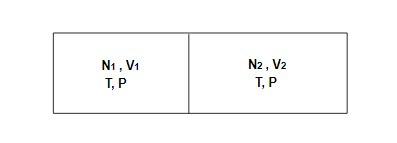The entropy of the system is a measure of the disorder of the gas. We know, for the entropy of a classical ideal gas is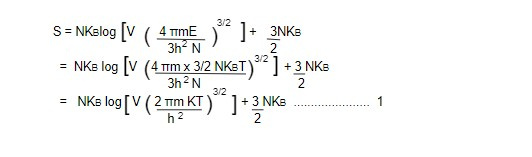Consider two perfect gas N1 molecules of one gas confined in volume V1 and other gas of N2 molecules confined in volume V2. Let two system initially are in same temperature (T) and pressure P separated by the partition as shown in above figure.

The entropy of each of the system is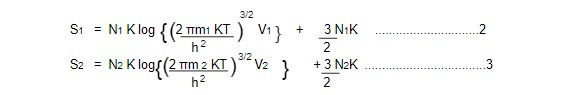When gases are allowed to mix then after mixing the volume becomes (V1+V2) and the total molecules becomes (N1+N2). The temperature remains constant after mixing so the entropy after mixing isThus, the change in entropy when they are mixed,

△S = S12 - (S1+S2)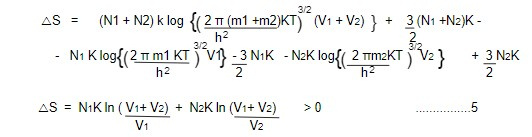This equation 5 is clearly a positive quantity. This result suggests when two different gases are mixed together even at same temperature and pressure, the entropy of a system increases. This is in good agreement for the irreversible process.

But if the process is reversible equation 5 doesn't hold in good agreement. This is Gibb's paradox.

This paradox was resolved by Gibb's in empirical fashion by postulating that we have made an error in calculating Σ (E) (phase space volume in the energy O to E). One has to regard all the identical molecules as indistinguishable. Gibb's assumed that the correct answer is N! smaller that we thought it was. The division of Σ (E) by N! accounts for indistinguishability of gas molecules which was understood only after the development of quantum mechanical idea. By this assumptions eq 1 becomes,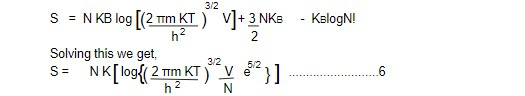This eq 6 is known as Sackur Tetrode equation for indistinguishable gas.

The Sackur-Tetrode equation is important in the field of thermodynamics because it provides a quantitative description of the entropy of an ideal gas, which is a fundamental property of a thermodynamic system. The equation is also useful in the calculation of various thermodynamic quantities, such as the internal energy and free energy of an ideal gas.

Let us consider N1 particles confined in volume V1 are mixed with N2 molecules confined in volume V2 at same initial temperature and pressure. The entropy of each system is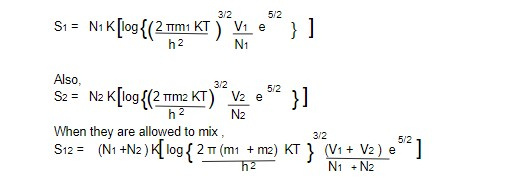Now, change in entropy of system,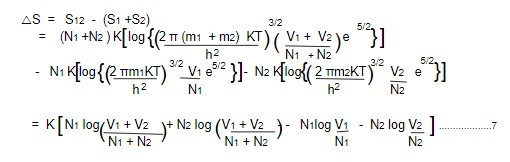The equation 7 is same as before equation 5 but if two volume of same kind of gas at same temperature and pressure are mixed together. Therefore for reversible process △S = 0 because

N1/V1 = N2/V2 = N1+ N2 /(V1+V2) [as V/N - specific volume which is same before and after mixing]

The resolution of Gibbs' paradox has important implications in the field of statistical mechanics, which deals with the behavior of systems consisting of many particles. Understanding the paradox and its resolution is essential for correctly calculating the thermodynamic properties of mixtures of gases.

One of the key applications of the resolution to Gibbs' paradox is in the design and operation of various industrial processes, such as in the chemical and petrochemical industries. For example, the resolution of the paradox can help engineers and scientists to accurately predict the thermodynamic behavior of gas mixtures in reactors and pipelines, which is crucial for optimizing process efficiency and avoiding accidents.

Furthermore, the resolution of Gibbs' paradox is also relevant to the study of complex biological systems, such as the behavior of proteins and other macromolecules. The ability to accurately calculate the thermodynamic properties of such systems is essential for understanding their behavior and designing effective drugs and therapies.

Overall, the resolution of Gibbs' paradox is a fundamental concept in statistical mechanics that has many practical applications in various fields of science and engineering.

This note is taken from Statistical Mechanics, MSC physics, Nepal.

This note is a part of the Physics Repository.